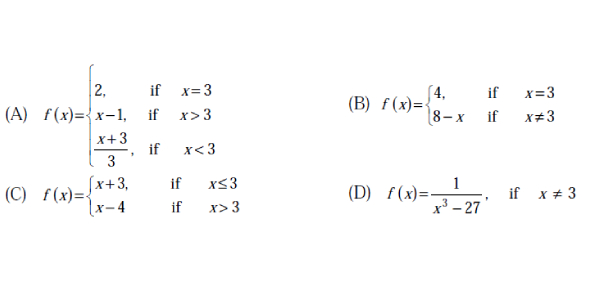# SSC CGL Exam Tier I: Numerical Ability Quiz!

50 Questions | Attempts: 2027Settings.

• 1.
In how many years will a sum of Rs. 800 at 10% per annum compound interest, compounded semi-annually becomes Rs. 926.10?
• A.
• B.
• C.
• D.
• 2.
Kamal defeats Bimal by 5 seconds in a 100 m race. If the speed of Kamal is 18 Kmph, then the speed of Bimal is
• A.

15.4 kmph

• B.

14.5 kmph

• C.

14.4 kmph

• D.

14 kmph

• 3.
A 240 m long train crosses a man walking along the line in the opposite direction at the rate of 3 kmph in 10 seconds. The speed of the train is
• A.

63 kmph

• B.

75 kmph

• C.

83.4 kmph

• D.

86.4 kmph

• 4.
A boatman rows 1 km in 5 minutes, along the stream, and 6 km in 1 hour against the stream. The speed of the stream is
• A.

3 kmph

• B.

6 kmph

• C.

10 kmph

• D.

12 kmph

• 5.
Atul can complete  of a work in 5 days and Bimal can complete  of the work in 10 days. In how many days both Atul and Bimal together can complete the work?
• A.

10

• B.
• C.
• D.
• 6.
7 men can complete a piece of work in 12 days. How many additional men will be required to complete double the work in 8 days?
• A.

28

• B.

21

• C.

14

• D.

7

• 7.
The average of odd numbers upto 100 is:
• A.

50.5

• B.

50

• C.

49.5

• D.

49

• 8.
One pipe fills a water tank three times faster than another pipe. If the two pipes together can fill the empty tank in 36 minutes, then how much time will the slower pipe alone take to fill the tank?
• A.

1 hour 21 minutes

• B.

1 hour 48 minutes

• C.

2 hours

• D.

2 hour 24 minutes

• 9.
In an examination, Amit scores 4 marks for every correct answer and loses 1 mark for every wrong answer. If he attempted all the 200 questions and scored, in all 200 marks. The number of questions, he answered correctly was:
• A.

82

• B.

80

• C.

68

• D.

60

• 10.
Two number are in the ratio 7 : 11. If 7 is added to each of the numbers, the ratio becomes 2 : 3. The smaller number is
• A.

39

• B.

49

• C.

66

• D.

77

• 11.
If Akash's income is 25% less than Bobby's income, by how much percent is Bobby's income more than that of Akash?
• A.

25

• B.

30

• C.
• D.
• 12.
The sixth term of the sequence 2, 6, 11, 17,………… is
• A.

24

• B.

30

• C.

32

• D.

36

• 13.
• A.
• B.
• C.
• D.
• 14.
A number, when divided by 136. Leaves remainder 36. If the same number is divided by 17, the remainder will be
• A.

9

• B.

7

• C.

3

• D.

2

• 15.
• A.
• B.
• C.

X

• D.
• 16.
A 4-digit number is formed by repeating a 2-digit number such as 1515, 3737, etc. Any number of this form is exactly divisible by
• A.

7

• B.

11

• C.

13

• D.

101

• 17.
• A.
• B.
• C.
• D.

• 18.
(0.1 x 0.01 x 0.001 X 107) is equal to
• A.

100

• B.
• C.
• D.

10

• 19.
If P and q represent digits, what is the possible maximum value of q in the statement 5p9 + 327 + 2q8 = 1114?
• A.

9

• B.

8

• C.

7

• D.

6

• 20.
• A.

4

• B.

5

• C.

8

• D.

15

• 21.
• A.
• B.
• C.
• D.
• 22.
• A.

1

• B.
• C.
• D.

10

• 23.
Out of six consecutive natural numbers, if the sum of first three is 27. What is the sum of the other three?
• A.

36

• B.

35

• C.

25

• D.

24

• 24.
The H.C.Fand L.C.M of two numbers are 12 and 336 respectively. If one of the numbers is 84, the other is
• A.

36

• B.

48

• C.

72

• D.

96

• 25.
The sum of two numbers is 36 and their H.C.F and L.C.M. are 3 and 105 respectively. The sum of the reciprocals oftwo numbers is
• A.
• B.
• C.
• D.

## Related TopicsBack to top
×

Wait!
Here's an interesting quiz for you.